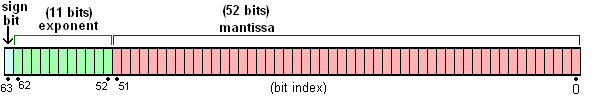# # JavaScript 关于 IEEE 754 双精度浮点数的实现

## # 双精度浮点数的存储

64 位比特又可分为三个部分：

• 符号位
• 指数位
• 尾数位 M：最后的 52 位是尾数（`mantissa`），超出的部分自动进一舍零### # 指数位

• 0x000: 即二进制的`00000000000`，用来代表带符号的 0（尾数为 0）或下溢数（尾数不为 0）。
• 0x7ff: 即二进制的`11111111111`，用来代表无穷大（尾数为 0）或NaN（尾数不为 0）。
• 其他: 即二进制的`00000000001`-`11111111110`代表 2 的`exponent-0x3ff`次方。

### # 尾数位

Mantissa * 2exponent

### # 总结1.10011001100110011001100110011001100110011001100110011【注意，第 53 为是 1】00...（1100无限循环）* 2-4

1.1001100110011001100110011001100110011001100110011010 * 2-4## # 计算误差

### # 安全整数

JavaScript 的`Number`构造函数上有如下两个静态属性:

• `Number.MAX_VALUE`: 常量，其值为`1.7976931348623157e+308`，表示 JavaScript 里所能表示的最大数值。
• `Number.MAX_SAFE_INTEGER`: 常量，其值为`9007199254740991`，即 253 - 1，表示 JavaScript 里所能表示的最大安全整数。

#### # 确定最大安全整数

1.{52个1} * 2exponent - 0x3ff

1.{52个1} * 22046 - 0x3ff = 1.{52个1} * 21023 = (253-1) * 21023 - 52 = (253-1) * 2971

253-3 {51个1}01 1.{50个1}01 * 252 `exponent`: 52 + 1023
`mantissa`: 50个1, 后跟01
（整数与浮点数一一对应，安全整数）
253-2 {51个1}10 1.{50个1}10 * 252 `exponent`: 52 + 1023
`mantissa`: 50个1, 后跟10
（整数与浮点数一一对应，安全整数）
253-1 {51个1}11 1.{50个1}11 * 252 `exponent`: 52 + 1023
`mantissa`: 50个1, 后跟11
（整数与浮点数一一对应，安全整数）
253 1{53个0} 1.{53个0} * 253 `exponent`: 53 + 1023
`mantissa`: 52 个 0
（只有 52 比特，最后一个 0 被省略了）
（两个整数对应一个浮点数，不安全整数）
253 + 1 1 {52个0} 1 1.{52个0}1 * 253 `exponent`: 53 + 1023
`mantissa`: 52 个 0
（只有 52 比特，最后一个 1 被省略了）
（两个整数对应一个浮点数，不安全整数）
253 + 2 1 {51个0} 10 1.{51个0}10 * 253 `exponent`: 53 + 1023
`mantissa`: 51 个 0, 1 个 1
（只有 52 比特，最后一个 0 被省略了）
（两个整数对应一个浮点数，不安全整数）
253 + 3 1 {51个0} 11 1.{51个0}11 * 253 `exponent`: 53 + 1023
`mantissa`: 51 个 0, 1 个 1
（只有 52 比特，最后一个 1 被省略了）
（两个整数对应一个浮点数，不安全整数）

``````Math.pow(2,53) === Math.pow(2,53) + 1 // true
``````
1

(253 ~ 254) 之间的数，只能精确表示 21 的倍数，即偶数

(254 ~ 255) 之间的数，只能精确表示 22 的倍数，即 4 的倍数

(255 ~ 256) 之间的数，只能精确表示 23 的倍数，即 8 的倍数

``````> Math.pow(2, 53)
9007199254740992
> Math.pow(2, 53) + 1
9007199254740992
> Math.pow(2, 53) + 2
9007199254740994
> Math.pow(2, 53) + 3
9007199254740996
> Math.pow(2, 53) + 4
9007199254740996
> Math.pow(2, 53) + 5
9007199254740996
> Math.pow(2, 53) + 6
9007199254740998
> Math.pow(2, 53) + 7
9007199254741000
> Math.pow(2, 53) + 8
9007199254741000
> Math.pow(2, 53) + 9
9007199254741000
> Math.pow(2, 53) + 10
9007199254741002
``````
1
2
3
4
5
6
7
8
9
10
11
12
13
14
15
16
17
18
19
20
21
22

Math.pow(2, 53) 9007199254740992 `exponent`: 52 + 1023，`mantissa`: 000...0000 （53 个 0） `mantissa`: 52 个 0
Math.pow(2, 53) + 1 9007199254740992 `exponent`: 52 + 1023，`mantissa`: 000...0001 （52 个 0 + 1） `mantissa`: 52 个 0
Math.pow(2, 53) + 2 9007199254740994 `exponent`: 52 + 1023，`mantissa`: 000...0010 （51 个 0 + 10） `mantissa`: 51 个 0 + 1
Math.pow(2, 53) + 3 9007199254740996 `exponent`: 52 + 1023，`mantissa`: 000...0011 （51 个 0 + 11） `mantissa`: 50 个 0 + 10
Math.pow(2, 53) + 4 9007199254740996 `exponent`: 52 + 1023，`mantissa`: 000...0100 （50 个 0 + 100） `mantissa`: 50 个 0 + 10
Math.pow(2, 53) + 5 9007199254740996 `exponent`: 52 + 1023，`mantissa`: 000...0101 （50 个 0 + 101） `mantissa`: 50 个 0 + 10
Math.pow(2, 53) + 6 9007199254740998 `exponent`: 52 + 1023，`mantissa`: 000...0110 （50 个 0 + 110） `mantissa`: 50 个 0 + 11
Math.pow(2, 53) + 7 9007199254741000 `exponent`: 52 + 1023，`mantissa`: 000...0111 （50 个 0 + 111） `mantissa`: 49 个 0 + 100
Math.pow(2, 53) + 8 9007199254741000 `exponent`: 52 + 1023，`mantissa`: 000...1000 （49 个 0 + 1000） `mantissa`: 49 个 0 + 100
Math.pow(2, 53) + 9 9007199254741000 `exponent`: 52 + 1023，`mantissa`: 000...1001 （49 个 0 + 1001） `mantissa`: 49 个 0 + 100
Math.pow(2, 53) + 10 9007199254741002 `exponent`: 52 + 1023，`mantissa`: 000...1010 （49 个 0 + 1010） `mantissa`: 49 个 0 + 101

### # 小数

#### # Number.EPSILON

ES6 在`Number`对象上面，新增一个极小的常量`Number.EPSILON`。根据规格，它表示 1 与大于 1 的最小浮点数之间的差。

1 的二进制科学计算法表示为：1.00...00 * 20，其中`mantissa`为 52 个 0 比 1 大的最小浮点数为：1.00...01 * 20，其中`mantissa`为 51 个 0 和 1 个 1。

#### # 小数误差#### # 0.1 + 0.2 = 0.30000000000000004

JavaScript 里关于数字的运算，都是先转为二进制，再转成二进制的科学计数法进行存储，再取出存储值转为二级制，最后进行运算。而在先存储再取出值的过程中，就可能丢失精度。

`0.1``0.2`存储后转为二进制的形式为：

`0.0001 1001 1001 1001 1001 1001 1001 1001 1001 1001 1001 1001 1001 1010` +

`0.0011 0011 0011 0011 0011 0011 0011 0011 0011 0011 0011 0011 0011 010` =

`0.0100 1100 1100 1100 1100 1100 1100 1100 1100 1100 1100 1100 1100 111`

#### # 为什么 x = 0.1 能得到 0.1

1.{51个0}1 * 20，（即`exponent`为十进制的`1023``mantissa`为二进制的`{51个0}1`），转换为十进制就是 20 + 2-52

``````0.1.toPrecision(21) // 0.100000000000000005551
0.2.toPrecision(21) // 0.200000000000000011102
0.3.toPrecision(21) // 0.299999999999999988898
0.4.toPrecision(21) // 0.400000000000000022204
0.5.toPrecision(21) // 0.500000000000000000000
0.6.toPrecision(21) // 0.599999999999999977796
0.7.toPrecision(21) // 0.699999999999999955591
0.8.toPrecision(21) // 0.800000000000000044409
0.9.toPrecision(21) // 0.900000000000000022204
``````
1
2
3
4
5
6
7
8
9

``````0.10000000000000000555.toPrecision(16) // 0.1000000000000000
``````
1

## # IEEE 754是按照什么规则来实现双精度浮点数的截断的？

`Number.EPSILON`一节我们知道，双精度浮点数的小数精度为 2-52，即 2.220446049250313e-16，但是在将双精度浮点数转换为十进制的数字字符串时，小数点后要保留多少位有效数字呢？我们先来看看浏览器控制台的一组计算及其返回：

``````0.1 + 0.2 = 0.30000000000000004     // 17 位有效数字
100.27 * 0.41 = 41.110699999999994  // 17 位有效数字
0.95 * 1 / 3 = 0.31666666666666665  // 17 位有效数字
300.73 - 300 = 0.7300000000000182   // 16 位有效数字
``````
1
2
3
4

The standard requires operations to convert between basic formats and external character sequence formats. Conversions to and from a decimal character format are required for all formats. Conversion to an external character sequence must be such that conversion back using round to even will recover the original number. There is no requirement to preserve the payload of a NaN or signaling NaN, and conversion from the external character sequence may turn a signaling NaN into a quiet NaN. The original binary value will be preserved by converting to decimal and back again using 17 decimal digits for binary64.

IEEE 754 规定，浮点数被转成十进制数字字符串，当这个字符串（使用 Round to Even 向偶舍入）转回浮点数时，必须要跟原来的数相同。对双精度浮点数来说，十进制字符串使用17位有效数字即可保存原始二进制值。

## # TODO

TODO: 如何在 Javascript 数字计算中保证精度正确？ number-precision

## # Reference

Last Updated: 7/5/2019, 9:43:03 AM